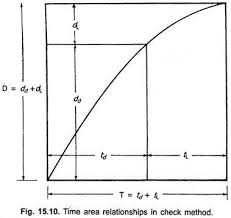## How to Calculate and Solve for Time Required to Irrigate | Irrigation Water RequirementThe image above represents time required to irrigate.

To compute for time required to irrigate, three essential parameters are needed and these parameters are Area of the Land (A), Depth of the Water to be Applied (dwand Discharge of the Stream (q).

The formula for calculating time required to irrigate:

t = A . dw / q

Where:

t = Time Required to Irrigate
A = Area of the Land
dw = Depth of the Water to be Applied
q = Discharge of the Stream

Let’s Solve an example;
Find the time required to irrigate when the area of the land is 14, the depth of the water to be applied is 20 and the discharge of the stream is 10.

This implies that;

A = Area of the Land = 14
dw = Depth of the Water to be Applied = 20
q = Discharge of the Stream = 10

t = A . dw / q
t = 14 . 20 / 10
t = 280 / 10
t = 28

Therefore, the time required to irrigate is 28.

Calculating for the Area of the Land when the Time Required to Irrigate, the Depth of the Water to be Applied and the Discharge of the Stream is Given.

A = t x q / dw

Where;

A = Area of the Land
t = Time Required to Irrigate
dw = Depth of the Water to be Applied
q = Discharge of the Stream

Let’s Solve an example;
Find the area of the Land when the time required to irrigate is 20, the depth of the water to be applied is 12 and the discharge of the stream is 3.

This implies that;

t = Time Required to Irrigate = 20
dw = Depth of the Water to be Applied = 12
q = Discharge of the Stream = 3

A = t x q / dw
A = 20 x 3 / 12
A = 60 / 12
A = 5

Therefore, the area of the land is 5.